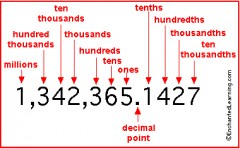# What Is 1-1/2 In Decimal Form?

There are two ways to look at this question. Firstly, you could mean what is one and a half in decimal terms, which is 1.5. You could alternatively mean what is one minus a half, which is a half, or 0.5.
Fractions (from the Latin 'fractus', meaning "broken") are numbers expressed as a ratio of two numbers. They are used as a comparison between parts and a whole. Fraction to decimal conversion is very simple mathematics. Common fractions, such as _ and _ consist of a numerator and a denominator. The top number is the numerator, and represents the part. The bottom is denominator and represents the whole.
Decimalisation was a later introduction, but operates along the same principles. For example, 0.7 as a fraction is 7/10. The reason for this is that the 7 is a single digit after the decimal point. A decimal that has only one digit after the point has a denominator of 10. If it has 2 digits it's 100. 3 digits and it's 1000. A simple trick is for every digit you should add a zero to a one for the denominator.
If a fraction doesn't immediately work on the basis on being immediately a part of 10, 100 or 1000 then you should look to alter the fraction. An example is 2/5. This doubles to 4/10, giving you 0.4. You could perhaps have 3/50. Doubling it gets you 6/100 = 0.06. In both the cases the rule applies; the denominator of 10 means you have just one digit post-decimal. The denominator of 100 means you have two.
thanked the writer.
1.5
thanked the writer.
1.5    one dot five. :) have a great day.
thanked the writer.1 1/2 = 1 5/10 = 1.5
1 - 1/2 = 1/2 = 5/10 = 0.5
thanked the writer.